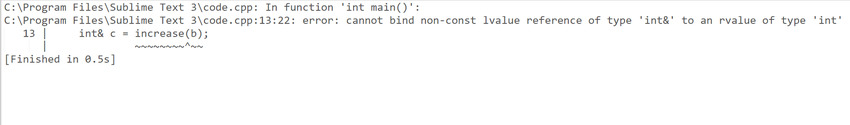Open in App
Not now

# Return type deduction in C++14 with Examples

• Difficulty Level : Expert
• Last Updated : 28 Jan, 2021

In this article, we will discuss Return Type Deduction in C++14. Using an auto return type in C++14, the compiler will attempt to deduce the return type automatically.

Program 1:

## C++14

 `// C++14 program to illustrate the``// return type deduction``#include ``using` `namespace` `std;`` ` `// Function to multiply the two``// numbers a and b``auto` `multiply(``int` `a, ``int` `b)``{`` ` `    ``// Return the product``    ``return` `a * b;``}`` ` `// Driver Code``int` `main()``{``    ``int` `a = 4, b = 5;`` ` `    ``// Function Call``    ``cout << multiply(a, b);`` ` `    ``return` `0;``}`

Output:

```20
```

Explanation: In the above program, the multiply(int a, int b) function the compiler will perform the multiplication. As the passed parameters 4 and 5, the compiler will return 20 and since its data type is an integer, the compiler will deduce the type as integer automatically and will return 20 as an integer.

Program 2:

## C++14

 `// C++ 14 program to illustrate the``// return type deduction``#include ``using` `namespace` `std;`` ` `// Function to increment the``// value of a``auto` `increase(``int``& a)``{``    ``// Increment a``    ``a++;`` ` `    ``// Return the updated value``    ``return` `a;``}`` ` `// Driver Code``int` `main()``{``    ``int` `b = 10;`` ` `    ``// Function Call``    ``int``& c = increase(b);`` ` `    ``// Print the value b and c``    ``cout << b << c;``    ``return` `0;``}`

Output:Explanation: As it can be seen that in the above program compiler is showing an error. This is because in the function increase(int &a), the compiler will return 11 and since its type is an integer, the compiler will deduce its type as integer and return it, but in main, we are assigning an integer value to an integer reference variable c, that’s why it was showing error.

Now, the above problem can be fixed in two ways:

## C++14

 `// C++14 program to illustrate return``// type deduction using auto&``#include ``using` `namespace` `std;`` ` `// Function to increment the value``// of a and return the updated value``auto``& increase(``int``& a)``{``    ``a++;``    ``return` `a;``}`` ` `// Driver Code``int` `main()``{``    ``int` `b = 10;`` ` `    ``// Function Call``    ``int``& c = increase(b);``    ``cout << b << ``'\n'``         ``<< c;`` ` `    ``return` `0;``}`

Output:

```11
11
```

## C++14

 `// C++14 program to illustrate return``// type deduction using decltype()``#include ``using` `namespace` `std;`` ` `// Function that increments the value``// of a and return the updated value``decltype``(``auto``) increase(``int``& a)``{``    ``a++;``    ``return` `a;``}`` ` `// Driver Code``int` `main()``{``    ``int` `b = 10;`` ` `    ``// Function Call``    ``int``& c = increase(b);`` ` `    ``cout << b << ``'\n'``         ``<< c;`` ` `    ``return` `0;``}`

Output:

```11
11
```

My Personal Notes arrow_drop_up